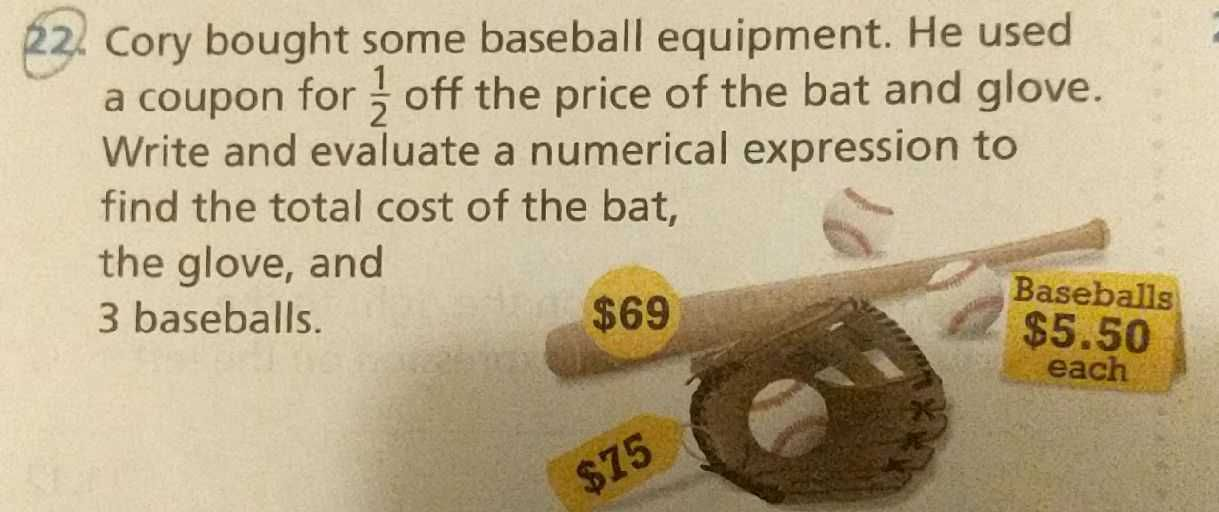### ¿Todavía tienes preguntas de matemáticas?

Pregunte a nuestros tutores expertos
Algebra
Pregunta22. Cory bought some baseball equipment. He used a coupon for $$\frac { 1 } { 2 }$$ off the price of the bat and glove. Write and evaluate a numerical expression to find the total cost of the bat, the glove,and $$3$$ baseballs.

(69+75+5.5$$\times 3$$)$$\times \frac{1}{2}$$=80.25